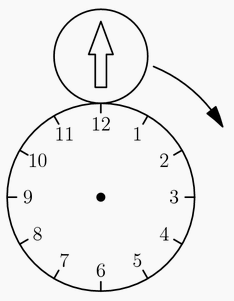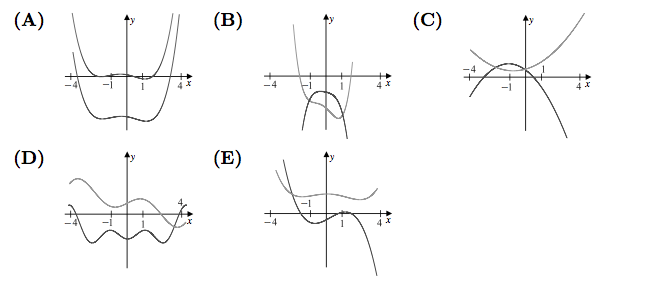###### back to index | new

If $x^m - y^n = (x+y^2)(x-y^2)(x^2+y^4)$, find the value of $m+n$.

The diagram below shows the circular face of a clock with radius $20$ cm and a circular disk with radius $10$ cm externally tangent to the clock face at $12$ o'clock. The disk has an arrow painted on it, initially pointing in the upward vertical direction. Let the disk roll clockwise around the clock face. At what point on the clock face will the disk be tangent when the arrow is next pointing in the upward vertical direction?Orvin went to the store with just enough money to buy $30$ balloons. When he arrived, he discovered that the store had a special sale on balloons: buy $1$ balloon at the regular price and get a second at $\frac{1}{3}$ off the regular price. What is the greatest number of balloons Orvin could buy?

Let $a$ and $b$ be relatively prime integers with $a>b>0$ and $\frac{a^3-b^3}{(a-b)^3}$ = $\frac{73}{3}$. What is $a-b$?

Rectangle $ABCD$ has $AB = 6$ and $BC = 3$. Point $M$ is chosen on side $AB$ so that $\angle AMD = \angle CMD$. What is the degree measure of $\angle AMD$?

At Jefferson Summer Camp, $60\%$ of the children play soccer, $30\%$ of the children swim, and $40\%$ of the soccer players swim. To the nearest whole percent, what percent of the non-swimmers play soccer?

Convex quadrilateral $ABCD$ has $AB = 9$ and $CD = 12$. Diagonals $AC$ and $BD$ intersect at $E$, $AC = 14$, and $\triangle AED$ and $\triangle BEC$ have equal areas. What is $AE$?

A bug starts at one vertex of a cube and moves along the edges of the cube according to the following rule. At each vertex the bug will choose to travel along one of the three edges emanating from that vertex. Each edge has equal probability of being chosen, and all choices are independent. What is the probability that after seven moves the bug will have visited every vertex exactly once?

What value of $a$ satisfies $27x^3 - 16\sqrt{2}=(3x-2\sqrt{2})(9x^2 + 12x\sqrt{2}+a)$?

If $-1 < a < b < 0$, then which relationship below holds? $(A)\quad a < a^3 < ab^2 < ab \qquad (B)\quad a < ab^2 < ab < a^3 \qquad (C)\quad a< ab < ab^2 < a^3 \qquad (D) a^3 < ab^2 < a < ab$

If the sum of all coefficients in the expanded form of $(3x+1)^n$ is $256$, find the coefficient of $x^2$.

The nonzero coefficients of a polynomial $P$ with real coefficients are all replaced by their mean to form a polynomial $Q$. Which of the following could be a graph of $y = P(x)$ and $y = Q(x)$ over the interval $-4\leq x \leq 4$?Several sets of prime numbers, such as $\{7,83,421,659\}$ use each of the nine nonzero digits exactly once. What is the smallest possible sum such a set of primes could have?

Find the remainder when you divide $(x^{81} + x^{49} + x^{25} + x^9 + x)$ by $(x^3 - x)$.

Find the sum of all the coefficients of $(x_1+x_2+ \cdots+x_{2016})^{2016}$.

For a certain positive integer $n$ less than $1000$, the decimal equivalent of $\frac{1}{n}$ is $0.\overline{abcdef}$, a repeating decimal of period of $6$, and the decimal equivalent of $\frac{1}{n+6}$ is $0.\overline{wxyz}$, a repeating decimal of period $4$. In which interval does $n$ lie?

In $\triangle ABC$ let $I$ be the center of the inscribed circle, and let the bisector of $\angle ACB$ intersect $\overline{AB}$ at $L$. The line through $C$ and $L$ intersects the circumscribed circle of $\triangle ABC$ at the two points $C$ and $D$. If $LI=2$ and $LD=3$, then $IC= \frac{p}{q}$, where $p$ and $q$ are relatively prime positive integers. Find $p+q$.

A strictly increasing sequence of positive integers $a_1$, $a_2$, $a_3$, $\cdots$ has the property that for every positive integer $k$, the subsequence $a_{2k-1}$, $a_{2k}$, $a_{2k+1}$ is geometric and the subsequence $a_{2k}$, $a_{2k+1}$, $a_{2k+2}$ is arithmetic. Suppose that $a_{13} = 2016$. Find $a_1$.

For polynomial $P(x)=1-\dfrac{1}{3}x+\dfrac{1}{6}x^{2}$, define $Q(x)=P(x)P(x^{3})P(x^{5})P(x^{7})P(x^{9})=\displaystyle\sum_{i=0}^{50} a_ix^{i}$. Find the value of $\displaystyle\sum_{i=0}^{50} |a_i|$.

Find the value of $$\binom{n}{0}-\binom{n}{1}+\binom{n}{2}-\binom{n}{3}+\cdots +(-1)^n\binom{n}{n}$$

Find $a$ and $b$ so that $(x-1)^2$ divides $ax^4 + bx^3+1$.

Find all pairs of real numbers $a, b$, such that the polynomial $$p(x)=(a+b)x^5 + abx^2 +1$$ is divisible by $x^2 - 3x+2$.

Let $n$ be a positive integer, and for $1\le k\le n$, let $S_k$ be the sum of the products of $1, \frac{1}{2}, \cdots, \frac{1}{n}$, taken $k$ a time ($k^{th}$ elementary symmetric polynomial). Find $S_1 + S_2 + \cdots +S_n$.